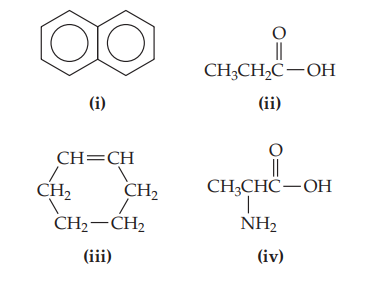×
Get Full Access to Chemistry: The Central Science - 14 Edition - Chapter 24 - Problem 24.3
Get Full Access to Chemistry: The Central Science - 14 Edition - Chapter 24 - Problem 24.3

×ISBN: 9780134414232 1274

## Solution for problem 24.3 Chapter 24

Chemistry: The Central Science | 14th Edition

• Textbook Solutions
• 2901 Step-by-step solutions solved by professors and subject experts
• Get 24/7 help from StudySoup virtual teaching assistantsChemistry: The Central Science | 14th Edition

4 5 1 337 Reviews
10
0
Problem 24.3

(b) Which of these molecules is aromatic?

(c) Which of these molecules most readily undergoes a substitution reaction? [Section 24.3]Step-by-Step Solution:
Step 1 of 3

R=clockwise S=counterclockwise *for a tetrahedral 3D stereoformula -never two lines 180˚ -never all wedges -never all dashes Chiral=not superimposable Achiral=superimposable;if a molecule has a plane of symmetry and is superimposable *NOTE:be able to determine if the structure is R or S *NOTE:meso=more than one chirality center but achiral overall Optical activity=ability of a chiral substance to rotate plane-polarized light (ppl) -measured by a polarimeter l=levorotatory=counterclockwise rotation of ppl (-) d=dextrorotatory=clockwise rotation of ppl (+) =observed angle of rotation Speciﬁc rotation=physical property of a chiral compound Optically pure/homochiral/enantiomers=only one pure enantiomer in the sample racemic mixture=50% (-) enantiomer and 50% (+) enantiomer;optically impure *NOTE:The R-enantiomers can be (+) or (-) and then whatever R is,S will be the opposite sign. Cahn-Ingold-Prelog Rules for R,S Notation 1. Higher atomic mass takes precedence 2. Find point of ﬁrst difference 3. Work outward from point of attachment 4. Evaluate substituent atoms one-by-one 5. Multiply bonded is considered a replicated substituent Fischer Projections=horizontal lines are wedges and vertical lines are dashed wedges Carbohydrates=Cn(H20)n glycerol (glycerin) = OlderTerminology D=(+) L=(-) *NOTE:memorize aldoses on slide 4-14 Epimers=stereoisomers with more than one chiral center and the conﬁguration of o

Step 2 of 3

Step 3 of 3

##### ISBN: 9780134414232

The answer to “?(a) Which of these molecules most readily undergoes an addition reaction? (b) Which of these molecules is aromatic? (c) Which of these molecules most readily undergoes a substitution reaction? [Section 24.3]” is broken down into a number of easy to follow steps, and 31 words. This textbook survival guide was created for the textbook: Chemistry: The Central Science, edition: 14. Chemistry: The Central Science was written by and is associated to the ISBN: 9780134414232. The full step-by-step solution to problem: 24.3 from chapter: 24 was answered by , our top Chemistry solution expert on 10/03/18, 06:29PM. Since the solution to 24.3 from 24 chapter was answered, more than 204 students have viewed the full step-by-step answer. This full solution covers the following key subjects: . This expansive textbook survival guide covers 29 chapters, and 2820 solutions.

## Discover and learn what students are asking

Calculus: Early Transcendental Functions : Slope Fields and Eulers Method
?In Exercises 1-8, verify the solution of the differential equation. Solution D

Chemistry: The Central Science : Liquids and Intermolecular Forces
?The phase diagram for neon is Use the phase diagram to answer the following

Statistics: Informed Decisions Using Data : Comparing Three or More Means (One-Way Analysis of Variance)
?The variability among the sample means is called ____________ sample variability, and the variability of each sample is the sample variability.

Statistics: Informed Decisions Using Data : Testing the Significance of the Least-Squares Regression Model
?Tar and Nicotine Every year the Federal Trade Commission (FTC) must report tar and nicotine levels in cigarettes to Congress. Tar and nicotine levels

Unlock Textbook Solution